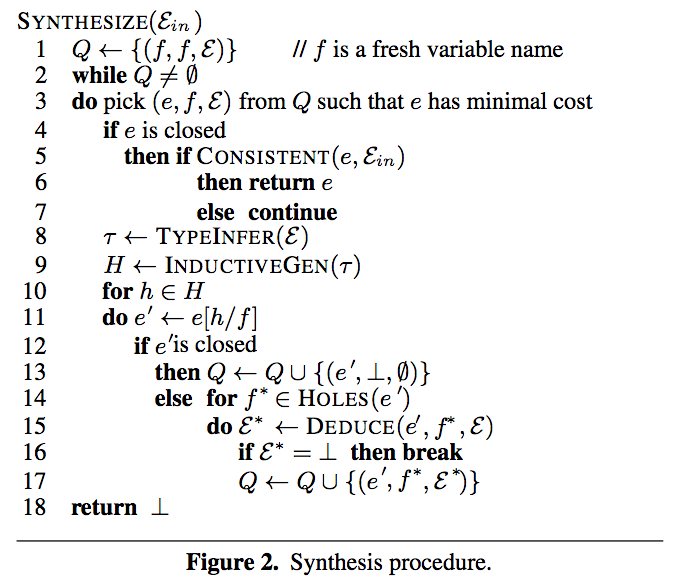# "Synthesizing Data Structure Transformations from Input-Output Examples"

• The paper presents a method to synthesize functional programs that transform recursive data structures (e.g., lists, trees)

• Examples: see Figure 6 (e.g., join, cprod)
• essentially shows how one can orchestrate a list of operators to generate the desired program
• Three techniques

• type-aware inductive generalization
• purpose: create hypotheses that represent some or all properties of the target program
• can be open -> contains "holes"
• can be closed -> if is consistent with examples, this is the program
• input: inferred types from examples
• use types -> prune the search space
• output: a stream of hypotheses (closed and open) that match types
• how:
• For open hypothesis, application of a higher-order combinator (Figure 3) to set of (known or unknown) arguments (use inferred types of examples to guide the selection of combinator)
• For close hypothesis, recursive procedure like enumerate search
• deduction
• purpose: solve unknown functions in hypotheses
• Two techniques:
• Refutation: use counter-example to reject hypotheses
• Example inference: uses properties of combinators to infer new examples on unknown functions
• best-first enumerate search
• weighted BFS idea -> use priority queue
• weight created from cost model: simple is better (avoid degenerate case: prog packed with if-blocks)
• Main algorithm• a priority queue $$Q$$ of subtasks $$(e, f, \mathcal{E})$$
• $$e$$: a hypothesis
• $$f$$: a hole in hypothesis
• $$\mathcal{E}$$: a set of examples
• pop the head of queue and obtain a subtask to work on
• if $$e$$ is closed, checking agains input examples
• ok -> got a solution; discard otherwise
• if $$e$$ is open
• synthesize $$f$$ in $$e$$
• infer type of $$f$$ from $$\mathcal{E}$$
• use inductive generalization to create a stream of hypotheses $$H$$ for $$f$$
• replace $$f$$ with each $$h \in H$$ to obtain a set of new hypotheses. Say one of them is $$e'$$
• if $$e'$$ is closed -> put $$(e', \perp, \emptyset)$$ as a subtask to queue
• if $$e'$$ is open
• new subtask for each hole $$f^*$$ in $$e'$$
• uses deduction on $$f^*$$ to create new examples $$\mathcal{E^*}$$ or refute
• add $$(e', f^*, \mathcal{E^*})$$ as a subtask if not rejected